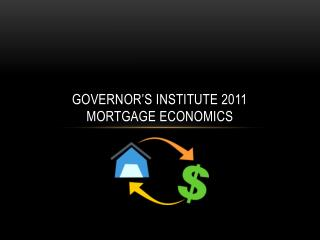DownloadDownload PresentationGovernor’s Institute 2011 Mortgage Economics

# Governor’s Institute 2011 Mortgage Economics

Télécharger la présentation## Governor’s Institute 2011 Mortgage Economics

- - - - - - - - - - - - - - - - - - - - - - - - - - - E N D - - - - - - - - - - - - - - - - - - - - - - - - - - -
##### Presentation Transcript

1. Governor’s Institute 2011Mortgage Economics

2. Interest Formulas (simple and compound) • Simple: = P + Pnr = original + interest • Compound: or discrete continuous

3. Example… A proud grandfather starts an college fund investment account for a newborn child. If the account pays a 6% annual rate with quarterly compounding, how much will the \$25,000 initial deposit be worth in 18 years when the child starts at UVM? P = \$25,000, r = 0.06, n = 4 & t = 18

4. Therefore… Now, what if we knew that the cost was going to be \$100,000? Could we solve for what the initial investment would need to be?

5. \$34,233.00 = P So we can see what an investment can grow to over time and we can solve for how much we need to start with in order to achieve some target amount in the future.

6. How do we sum a geometric series? • What is a geometric series? or .

7. Part 1 - The balloon payment. If you borrow an amount of money for a thirty year period, and then agree to pay it all back at once, this is considered a balloon payment. It is the most primitive mortgage. In that case, we use the basic discrete compounding formula using monthly compounding.

8. Part 2 – systematic savings. Let’s say that we are going to pay a set amount of money every month for the next thirty years in order to match the balloon payment. We need to figure out how to calculate the future value of all of those payments. That’s a lot of computations – 360 to be precise. Specifically we need to compute the following: T = M+ M+…+ M Where M is the monthly payment amount.

9. Remembering our snazzy formula: We see that this is equal to , which is , or

10. Let’s do an example. A disciplined saver puts \$200 aside every month. If the money is invested in an account that pays 9% interest compounded monthly, how much money will there be in 45 years?

11. If we wanted to figure out how much we needed to put aside every month to get to a particular amount, we could algebraically manipulate the equation to solve for M, given all the other variables.

12. To complete the mortgage formula, we realize that the balloon payment is the desired total that we want to systematically save up to in thirty years. Thus the formula is

13. So let’s look at a 30 year mortgage where we are borrowing \$100,000 and the prevailing interest rate is 6% annual rate with monthly compounding.

14. Could we find our budget? If we knew what we could afford for a monthly payment, could we determine what mortgage we could afford? Could we go in the other direction? • It is generally recognized that the feasible cutoff for household income to go to housing payment is 28%. Given a chosen house, can you determine what annual salary you’ll need to earn?